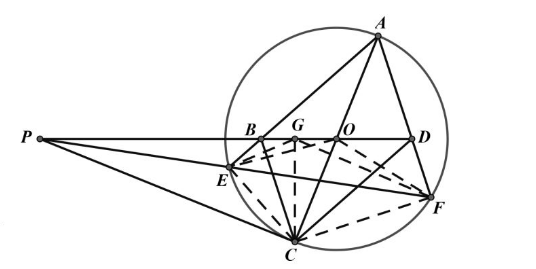【答案】 证明: 过 $C$ 作 $C G \perp P O$ 于 $G$,

$E 、 B 、 G 、 C$ 四点共圆

$P C$ 是 $\odot O$ 切线, $P C^2=P E \cdot P F$

$\therefore P E \cdot P F=P G \cdot P O$,
$\therefore E 、 G 、 O 、 F$ 四点共圆。 $\therefore \angle O G F$
$=\angle O E F, \angle B G E=\angle O E F, \quad \therefore \angle$
$O G F=\angle B G E$

$\therefore A F / / B C, A E / / C D, \therefore$ 四边形 $A B C D$ 是平行四边形。